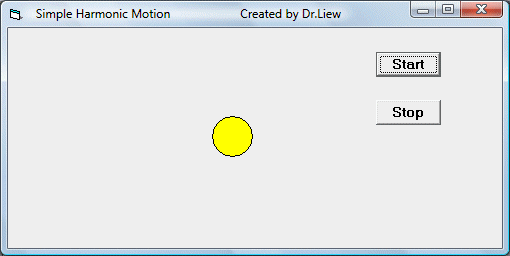# Simple Harmonic Motion Simulation Program

Simple harmonic motion is the motion of a simple harmonic oscillator. The motion is periodic, as it repeats itself at standard intervals in a specific manner with constant amplitude. It is characterized by its amplitude, its period(the time for a single oscillation), its frequency(the number of cycles per unit time), and its phase(which determines the starting point on the sine wave). The period, and its inverse the frequency, are constants determined by the overall system, while the amplitude and phase are determined by the initial conditions (position and velocity)of that system. (Wikipedia, 2008).

The equation of a simple harmonic motion is:

x=Acos(2pft+f),

where x is the displacement, A is the amplitude of oscillation, f is the frequency, t is the elapsed time, and f is the phase of oscillation.

To create a simple model of simple harmonic motion in VB6 , use the equation x=Acos(wt), and assign a value of 500 to A and a value of 50 to w.

In this program, you have to insert a shape and set it to be a circle in the properties windows. Next, insert two command buttons and change the captions to start and stop respectively. Finally, insert a timer and set its interval to be 1000 and disabled it at start up. We can use the move method  to move the shape1 object whose path is determined by  the formula  x = 500 * Cos(50 * t) . When you run the program, the will move in an oscillating motion as shown in the figure below:

### The Interface### The Code

Dim t As Integer

Private Sub cmd_Start_Click()

Timer1.Enabled = True

End Sub

Private Sub cmd_Stop_Click()
Timer1.Enabled = False

End Sub

Private Sub Timer1_Timer()
t = t + 1
x = 500 * Cos(50 * t)
Shape1.Move Shape1.Left + x
End Sub